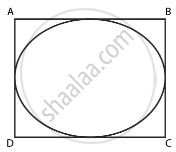# Is the area of the circle inscribed in a square of side a cm, πa2 cm2? Give reasons for your answer. - Mathematics

MCQ
True or False

Is the area of the circle inscribed in a square of side a cm, πa2 cm2? Give reasons for your answer.

• True

• False

#### Solution

This statement is False.

Explanation:

Let a be the side of square.

We are given that the circle is inscribed in the square.Diameter of circle = Side of square = a

Radius of the circle = a/2

Area of the circle = πr2 = π(a/2)^2 = (πa^2)/4 cm2

Hence, area of the circle is (πa^2)/4 cm2

`Thus the area of the circle inscribed in a square of side a cm is not a2 cm2

Concept: Area of Circle
Is there an error in this question or solution?

#### APPEARS IN

NCERT Mathematics Exemplar Class 10
Chapter 11 Area Related To Circles
Exercise 11.2 | Q 1 | Page 122

Share